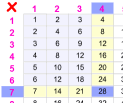# Division

### Example: there are 12 chocolates, and 3 friends want to share them, how do they divide the chocolates?12 Chocolates12 Chocolates Divided by 3

Answer: 12 divided by 3 is 4. They get 4 each.

## Symbols

÷  /

We use the ÷ symbol, or sometimes the / symbol to mean divide:

 12 ÷ 3 = 4 12 / 3 = 4

Let's use both symbols here so we get used to them.

## More Examples

Here are some more examples:

images/divide-simple.js

## Opposite of Multiplying

Division is the opposite of multiplying. When we know a multiplication fact we can find a division fact:

Example: 3 × 5 = 15, so 15 / 5 = 3.

Also 15 / 3 = 5.

Why? Well, think of the numbers in rows and columns like in this illustration:Multiplication...   ...Division
3 groups of 5 make 15...   ...so 15 divided by 3 is 5

and also:

5 groups of 3 make 15...   ...so 15 divided by 5 is 3.

So there are four related facts:

• 3 × 5 = 15
• 5 × 3 = 15
• 15 / 3 = 5
• 15 / 5 = 3

### Example: What is 28 ÷ 7 ?Searching around the multiplication table we find that 28 is 4 × 7, so 28 divided by 7 must be 4.

Answer: 28 ÷ 7 = 4

## Names

There are special names for each number in a division:

dividend ÷ divisor = quotient

### Example: in 12 ÷ 3 = 4:

• 12 is the dividend
• 3 is the divisor
• 4 is the quotient

## But Sometimes It Does Not Work Perfectly!

Sometimes we cannot divide things up exactly ... there may be something left over.

### Example: There are 7 bones to share with 2 pups.

But 7 cannot be divided exactly into 2 groups,
so each pup gets 3 bones,
but there will be 1 left over:We call that the Remainder.